### IMO Shortlist 1996 problem G7

Kvaliteta:
Avg: 0,0
Težina:
Avg: 9,0
Let$ABC$ be an acute triangle with circumcenter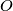$O$ and circumradius$R$.$AO$ meets the circumcircle of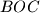$BOC$ at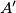$A'$,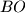$BO$ meets the circumcircle of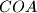$COA$ at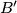$B'$ and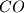$CO$ meets the circumcircle of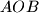$AOB$ at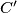$C'$. Prove that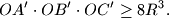Sorry if this has been posted before since this is a very classical problem, but I failed to find it with the search-function.
Izvor: Međunarodna matematička olimpijada, shortlist 1996